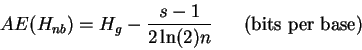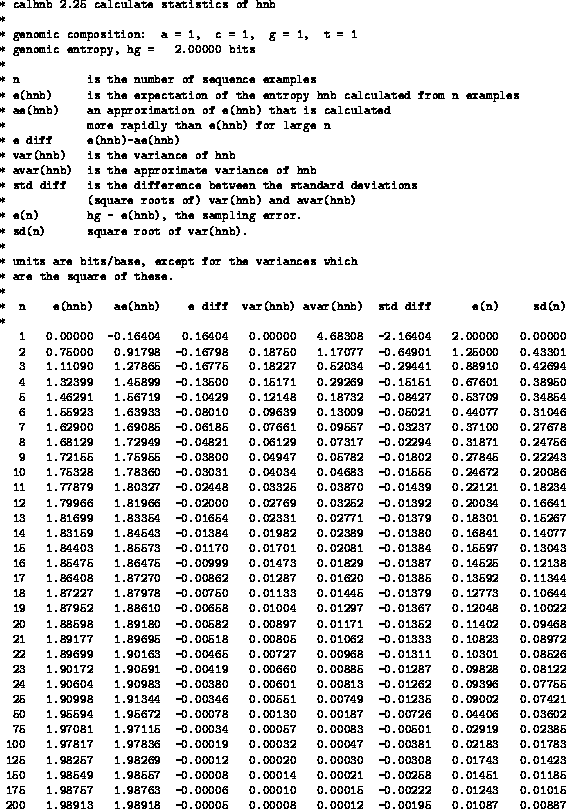>Next: (c) Use of the Up: APPENDIX Previous: (a) Exact method

## (b) Approximate method

The second method to calculate the sampling error correction is from Miller (1955) and Basharin (1959) who derived an approximation for the expectation of a sampled uncertainty, AE(Hnb), that is good for large n:(16)

where s, the number of symbols, is 4 for mononucleotides. Fig. 4 shows E(Hnb) and AE(Hnb) for several values of n. This table9 helps one to choose between AE(Hnb)(a computationally cheap estimate that is inaccurate for small n but accurate for large n) and E(Hnb) (an exact calculation that is computationally costly for large n). We use AE(Hnb) above n=50 because the cumulative difference between E(Hnb) and AE(Hnb)in a site 100 positions wide would be at most 0.078 bits. The exact E(Hnb) is used for n less than or equal to 50 since its computation is rapid in this range.Next: (c) Use of the Up: APPENDIX Previous: (a) Exact method
Tom Schneider
2002-10-16